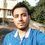# $\displaystyle \sum_{n_1=1}^\infty \sum_{n_2=1}^\infty \cdots \sum_{n_k=1}^\infty (-1)^{n_1+n_2+\cdots+n_k}\dfrac{H_{n_1+n_2+\cdots+n_k}}{n_1 n_2 n_3 \cdots n_k}$

\displaystyle \begin{aligned} &\sum_{n_1=1}^\infty \sum_{n_2=1}^\infty \cdots \sum_{n_k=1}^\infty (-1)^{n_1+n_2+\cdots+n_k}\dfrac{H_{n_1+n_2+\cdots+n_k}}{n_1 n_2 n_3 \cdots n_k} \\ &= (-1)^{k} \sum_{n=1}^{k} \binom{k}{n} (\log 2)^{k-n} \left[(-1)^n n! \zeta(n+1)+2(-1)^{n-1}\sum_{r=0}^n \binom{n}{r}r! (\log 2)^{n-r} {\rm Li}_{n+1}\left(\dfrac12\right) \right]\end{aligned}

The above is the claim which is proved here after in this note.

Proof:

First note that,

$\displaystyle H_n=\int_0^1 \dfrac{1-x^n}{1-x}\; dx$

Substituting this into our sum $S$ we have,

\displaystyle \begin{aligned} S&= \int_0^1 \dfrac{\sum_{n_1,n_2,\cdots,n_k=1}^\infty \dfrac{(-1)^{n_1+n_2+\cdots+n_k}}{n_1 n_2\cdots n_k}-\sum_{n_1,n_2,\cdots,n_k=1}^\infty \dfrac{(-1)^{n_1+n_2+\cdots+n_k}x^{n_1+n_2+\cdots+n_k}}{n_1 n_2\cdots n_k}}{1-x}\; dx \\ &= (-1)^k \int_0^1 \dfrac{\log^k 2-\log^k (1+x)}{1-x}\; dx \\ &= (-1)^k \int_{1/2}^1 \dfrac{\log^k 2-\log^k (2x)}{1-x}\; dx \\ &= (-1)^{k} \sum_{n=1}^{k} \binom{k}{n} (\log 2)^{k-n} \int_{1/2}^1 \dfrac{\log^n x}{1-x}\; dx \end{aligned}

Now it remains to calculate ,

$\displaystyle \int_{1/2}^1 \frac{\log^n x}{1-x}\; dx$

To find this we evaluate a preliminary integral,

\displaystyle \begin{aligned} & \int_{1/2}^1 x^m \; dx = \dfrac{1}{m+1}-\dfrac{2^{-m}}{1+m} \\ & \int_{1/2}^1 x^m \log^n x \; dx = \dfrac{d^n}{dm^n}\left[\dfrac{1}{m+1}-\dfrac{2^{-m}}{1+m}\right] \\ & \int_{1/2}^1 x^m \log^n x \; dx = \dfrac{(-1)^n n!}{(m+1)^{n+1}}+2^{-m}(-1)^{n-1} \sum_{r=0}^n \binom{n}{r}\dfrac{(\log 2)^{n-r}r!}{(m+1)^{r+1}}\end{aligned}

Now coming back to the main integral,

\displaystyle \begin{aligned} \int_{1/2}^1 \frac{\log^n x}{1-x}\; dx &= \sum_{m=0}^\infty \int_{1/2}^1 x^m \log^n x \; dx \\ &= \sum_{m=0}^\infty \left[ \dfrac{(-1)^n n!}{(m+1)^{n+1}}+2^{-m}(-1)^{n-1} \sum_{r=0}^n \binom{n}{r}\dfrac{(\log 2)^{n-r}r!}{(m+1)^{r+1}}\right] \\ &= (-1)^n n! \zeta(n+1)+2(-1)^{n-1}\sum_{r=0}^n \binom{n}{r}r! (\log 2)^{n-r} {\rm Li}_{n+1}\left(\dfrac12\right) \end{aligned}

Putting this back,

$\displaystyle S = (-1)^{k} \sum_{n=1}^{k} \binom{k}{n} (\log 2)^{k-n} \left[(-1)^n n! \zeta(n+1)+2(-1)^{n-1}\sum_{r=0}^n \binom{n}{r}r! (\log 2)^{n-r} {\rm Li}_{n+1}\left(\dfrac12\right) \right]$4 years, 2 months ago

This discussion board is a place to discuss our Daily Challenges and the math and science related to those challenges. Explanations are more than just a solution — they should explain the steps and thinking strategies that you used to obtain the solution. Comments should further the discussion of math and science.

When posting on Brilliant:

• Use the emojis to react to an explanation, whether you're congratulating a job well done , or just really confused .
• Ask specific questions about the challenge or the steps in somebody's explanation. Well-posed questions can add a lot to the discussion, but posting "I don't understand!" doesn't help anyone.
• Try to contribute something new to the discussion, whether it is an extension, generalization or other idea related to the challenge.

MarkdownAppears as
*italics* or _italics_ italics
**bold** or __bold__ bold
- bulleted- list
• bulleted
• list
1. numbered2. list
1. numbered
2. list
Note: you must add a full line of space before and after lists for them to show up correctly
paragraph 1paragraph 2

paragraph 1

paragraph 2

[example link](https://brilliant.org)example link
> This is a quote
This is a quote
    # I indented these lines
# 4 spaces, and now they show
# up as a code block.

print "hello world"
# I indented these lines
# 4 spaces, and now they show
# up as a code block.

print "hello world"
MathAppears as
Remember to wrap math in $$ ... $$ or $ ... $ to ensure proper formatting.
2 \times 3 $2 \times 3$
2^{34} $2^{34}$
a_{i-1} $a_{i-1}$
\frac{2}{3} $\frac{2}{3}$
\sqrt{2} $\sqrt{2}$
\sum_{i=1}^3 $\sum_{i=1}^3$
\sin \theta $\sin \theta$
\boxed{123} $\boxed{123}$

Sort by:

Very nice!

- 3 years, 10 months ago

What? :/

- 4 years, 2 months ago

He is so good at calculus that once his name was I guess published at the american mathematics daily becoz he sent a solution to a research level problm

- 3 years, 11 months ago

WOAH!!! Seriously???! This is soo great!

- 2 years, 5 months ago

yep i know.. he is a genius.. he is running a Youtube Channel too at present, Name is ANS Academy

- 2 years, 5 months ago

DAMN!!! Mujhe to uss channel ke baare mein aaj pata chala!!! Btw, if you want some interesting youtube channels, try "Faculty of Khan"....!!

- 2 years, 5 months ago

bhai mera 2 marks se KVPY choot gaya

- 2 years, 4 months ago

Wait shitt!!!!! Abbe yaar......!!!! 2 MARKS??!!!!!! Chal still don't lose hope......tu JEE mein bhi agar top 250 mein rank le aaye to IISC ho jaata hai...!! So, best of luck for it!!!!!

P.S. Main to iss saal ke liye prepare kar rhaa hun.....But mera ek dost hai, uska 11th waala clear hogaya and he got AIR 72....!!! Uska to pakka ho jaayega IISC mein......But problem is..........Usse research mein BILKUL interest nhi hai!!!! XD!!!! Pata nhi kyon hote hain aise log.......!!! XD!! (No offense.....:P)

- 2 years, 4 months ago

True.... Mera ek dost hai.. uska bhi same situation. AIR 55 aya .... SX mei .. aur phir IIT mein CSE lega bolta hai

- 2 years, 4 months ago

lets see.. thanks

- 2 years, 5 months ago

Great! I guess this something that he found interesting and wanted to share (Though i understand nothing of it)

- 3 years, 11 months ago

Same reaction here buddy:) I need to improve my calculus skills

- 4 years, 2 months ago

So do I :) She made me look like a noob :/

- 4 years, 2 months ago

I hope the 'she' doesn't refer to me , I'm a he :p

Um... I really should learn how to identify he/she, for real XD

- 4 years ago